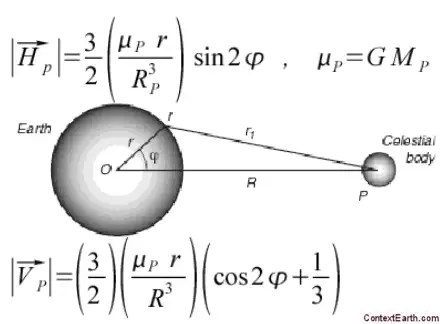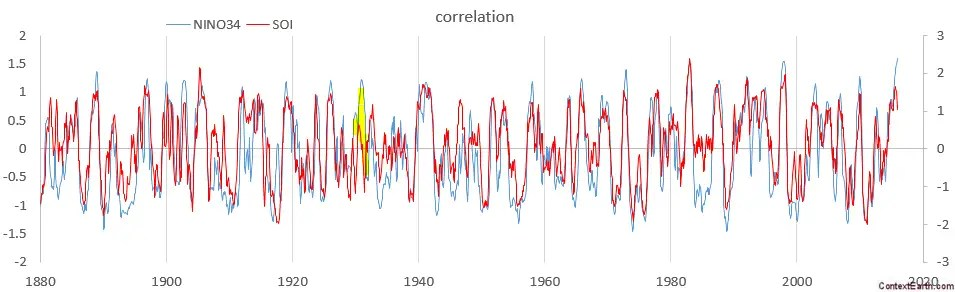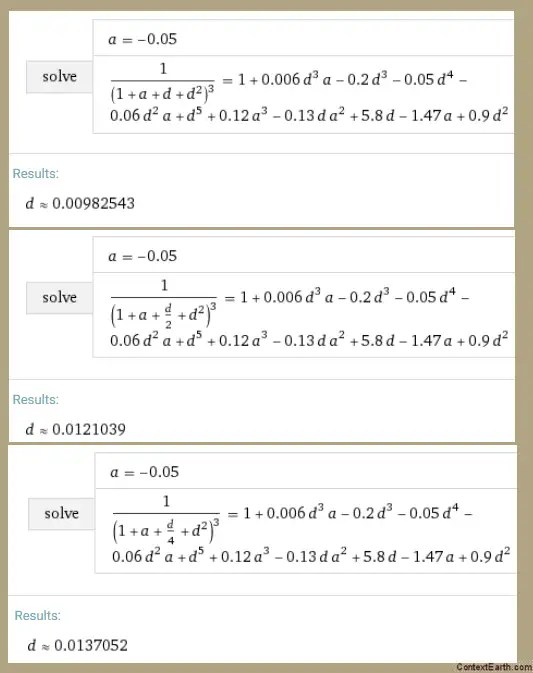# Reverse Engineering the Moon’s Orbit from ENSO Behavior

[mathjax]With an ideal tidal analysis, one should be able to apply the gravitational forcing of the lunar orbit1 and use that as input to solve Laplace’s tidal equations. This would generate tidal heights directly. But due to aleatory uncertainty with respect to other factors, it becomes much more practical to perform a harmonic analysis on the constituent tidal frequencies. This essentially allows an empirical fit to measured tidal heights over a training interval, which is then used to extrapolate the behavior over other intervals.  This works very well for conventional tidal analysis.

For ENSO, we need to make the same decision: Do we attempt to work the detailed lunar forcing into the formulation or do we resort to an empirical bottoms-up harmonic analysis? What we have being do so far is a variation of a harmonic analysis that we verified here. This is an expansion of the lunar long-period tidal periods into their harmonic factors. So that works well. But could a geophysical model work too?

We start with the lunar forcing, which in terms of the basic gravitational models, leads to a Newtonian inverse power law dependence. Below is a simplification of the model based on the geophysical geometry of the forcing. The gravitational forcing on the surface of the earth goes as $$~1/R^3$$, where R is the distance to the moon.Because the moon does change distance via the anomalistic cycle (a(t)), and as with ocean tides, this is further influenced by the draconic cycle (d(t)), we can make a substitution of :$R = R_0 + a(t) + d(t)$

or$R/R_0 = 1 + a(t)/R_0 + d(t)/R_0$

This is where we can start doing simplifications. Since a(t) and d(t) are both small with respect to $$R_0$$, we can expand this in a Taylor’s series about unity.$frac{1}{(R/R_0)^3} = 1 - 3 (a_R(t) + d_R(t)) + 6 (a_R(t) + d_R(t))^2 -10 (a_R(t) + d_R(t))^3 + ...$

where $$a_R(t)=a(t)/R_0, d_R(t)=d(t)/R_0$$

Further, since d(t) is strongly modulated by a fortnightly term in addition to the monthly term, this expands into a rich set of harmonics described by the following constituent factors (ignoring for the moment that the sine waves are further modulated by the synodic cycle)$d_R(t) = d_0 sin(omega_d t) + D_0 sin^2(omega_d t)$$a_R(t) = a_0 sin(omega_a t)$

This essentially explains why we have the terms broken out as described here with this essential set, where A is the anomalistic fundamental, and D is the draconic fundamental.$D, T, A, D^2, A^2, DA, D^2A, DA^2, D^2A^2$

and further refined here with this set$D, A, D^2, D^3, D^4, A^3, DA^2, DA$

These are essentially the first and second order terms which are selected based on trade-offs in how much they contribute without leading to over-fitting.

To the cubic power expansion, there are 15 terms to consider (not in any order)$D^3A, D^3, D^4, D^2A, D^6, D^5, D^4A, A^3, D^2A^2, DA^2, D, A, D^2, DA, A^2$

This is the working ENSO model fit:The small ~1 year region centered at 1931 is the primary mismatch between model and the NINO34 data. As described earlier, this error is also distinct and quantitatively resolved in the comparisons between the NINO34 and SOI measures.Whether that mismatch is a systemic measurement error or some real but unknown climatic or geophysical effect that occurred in 1931, it points to the difficulty of iteratively searching for an optimal model without over-fitting to potential non-ENSO related disturbances.

In any case, if we group the lunar factor terms which lead to the model fit and then solve for possible roots of a and d, which may reveal the original$1/(1 + a + d)^3 = f(a,d)$

we find this relation after first eliminating the weaker factors from the equation, and assuming that the monthly and fortnightly draconic terms have a 1:2 strength relationship:The role of the primary terms of D and A are more clear for large values but that is not the Taylor’s series range that we need to consider for small lunar perturbations. A linearization around the origin is where we can find how D and A relate.

So, if we choose a value for A around 0.05, and a range of monthly D and fortnightly D^2 values, we get these evaluations:Increasing A to a stronger value of -0.1Note that the draconic values are less than 0.1, which is within the constraints of the Taylor’s series expansion.  There are likely other constraints available, such as the LOD measure or another lunisolar invariant, which would make the solution less under-determined.

This is a promising approach and does demonstrate how well one can infer the moon’s orbit from the ENSO data, just as we can reverse engineer diurnal and semidiurnal tidal data to infer the strengths of the lunisolar factors.

## Footnotes

1 Not neglecting or forgetting the solar tidal gravitational forcing